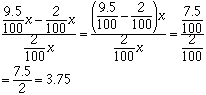Sender: Ann Allen Subject: percentage increase I am a a student in the 12th grade. I need to know how to calclulate the following: A percentage increase from 2.0% to 9.5% is what percentage? Hi Ann, If a quantity changes from a first value to second value then the percentage change is the difference, as a percentage of the first value. This sounds complicated. Let's look at an example    Suppose that you are working for me selling books. I am going to pay you 2% of the value of the books you sell. You sold \$1000.00 worth of books so I am to pay you 2% of \$1000, that is (2/100)x\$1000 = \$20.00. You did so well that I have decided to increase your commission by paying you 9.5% of the sales you make, that is 9.5% of \$1000 or (9.5/100)\$1000 = \$95.00.    Thus your salary has increased from \$20.00 to \$95.00, that is an increase of \$75.00. As a fraction of your starting salary this is 75/20 = 3.75 or 375%.    Notice that this percentage increase of 375% does not depend on the \$1000. If you had sold \$x worth of books then the percentage increase would beThat is 375%. Cheers, Harley

Go to Math Central